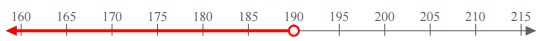# Writing an Inequality Given a Graph on the Number Line

In this lesson, given a graph on the number line, we write an inequality for the graph.

Rules to write an inequality given a graph on the number line.

For any variable x

• If the graph is a horizontal line starting from a point ‘a’ with an open circle and an arrow towards left, it is a less than inequality. x < a

• If the graph is a horizontal line starting from a point ‘a’ with a closed circle and an arrow towards left, it is a less than or equal to inequality. x ≤ a

• If the graph is a horizontal line starting from a point ‘a’ with an open circle and an arrow towards right, it is a greater than inequality. x > a

• If the graph is a horizontal line starting from a point ‘a’ with a closed circle and an arrow towards right, it is a greater than or equal to inequality. x ≥ a

Write an inequality for the graph on the number line given below.### Solution

Step 1:

From the given graph on the number line since the arrow is towards left and there is an open circle, it is a ‘less than’ inequality.

Step 2:

Since it is starting from 190 with an open circle, the inequality is x < 190

Write an inequality for the graph on the number line given below### Solution

Step 1:

From the given graph on the number line since the arrow is towards left and there is a closed circle, it is ‘less than or equal to’ inequality.

Step 2:

Since it is starting from −15 with a closed circle, the inequality is x ≤ −15